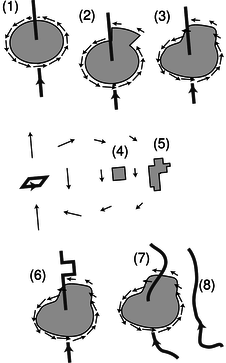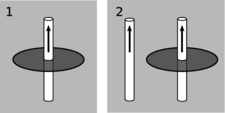$$\require{cancel}$$

11.3 Magnetic Fields by Ampère's Law

•• Contributed by Benjamin Crowell
• Professor (Physics) at Fullerton College

11.3.1 Ampère's law

As discussed at the end of subsection 11.2.4, our goal now is to find an equation for magnetism that, unlike the Biot-Savart law, will not end up being a dead end when we try to extend it to nonstatic situations.6 Experiments show that Gauss' law is valid in both static and nonstatic situations, so it would be reasonable to look for an approach to magnetism that is similar to the way Gauss' law deals with electricity.a / The electric field of a sheet of charge, and the magnetic field of a sheet of current.

How can we do this? Figure a, reproduced from page 664, is our roadmap. Electric fields spread out from charges. Magnetic fields curl around currents.b / A Gaussian surface and an Ampèrian surface.

In figure b/1, we define a Gaussian surface, and we define the flux in terms of the electric field pointing out through this surface. In the magnetic case, b/2, we define a surface, called an Ampèrian surface, and we define a quantity called the circulation, $$\Gamma$$ (uppercase Greek gamma), in terms of the magnetic field that points along the edge of the Ampèrian surface, c.c / The definition of the circulation, $$\Gamma$$.

We break the edge into tiny parts $$\mathbf{s}_j$$, and for each of these parts, we define a contribution to the circulation using the dot product of $$d\mathbf{s}$$ with the magnetic field:
$\begin{equation*} \Gamma = \sum \mathbf{s}_j\cdot\mathbf{B}_j \end{equation*}$

The circulation is a measure of how curly the field is. Like a Gaussian surface, an Ampèrian surface is purely a mathematical construction. It is not a physical object.

In figure b/2, the field is perpendicular to the edges on the ends, but parallel to the top and bottom edges. A dot product is zero when the vectors are perpendicular, so only the top and bottom edges contribute to $$\Gamma$$. Let these edges have length $$s$$. Since the field is constant along both of these edges, we don't actually have to break them into tiny parts; we can just have $$\mathbf{s}_1$$ on the top edge, pointing to the left, and $$\mathbf{s}_2$$ on the bottom edge, pointing to the right. The vector $$\mathbf{s}_1$$ is in the same direction as the field $$\mathbf{B}_1$$, and $$\mathbf{s}_2$$ is in the same direction as $$\mathbf{B}_2$$, so the dot products are simply equal to the products of the vectors' magnitudes. The resulting circulation is

\begin{align*} \Gamma &= |\mathbf{s}_1||\mathbf{B}_1|+|\mathbf{s}_2||\mathbf{B}_2| \\ &= \frac{2\pi k\eta s}{c^2}+\frac{2\pi k\eta s}{c^2} \\ &= \frac{4\pi k\eta s}{c^2} . \end{align*}

But $$\eta s$$ is (current/length)(length), i.e., it is the amount of current that pierces the Ampèrian surface. We'll call this current $$I_{through}$$. We have found one specific example of the general law of nature known as Ampère's law:

$\begin{equation*} \Gamma = \frac{4\pi k}{c^2}\,I_{through} \end{equation*}$

Positive and negative signsd / Positive and negative signs in Ampère's law.

Figures d/1 and d/2 show what happens to the circulation when we reverse the direction of the current $$I_{through}$$. Reversing the current causes the magnetic field to reverse itself as well. The dot products occurring in the circulation are all negative in d/2, so the total circulation is now negative. To preserve Ampère's law, we need to define the current in d/2 as a negative number. In general, determine these plus and minus signs using the right-hand rule shown in the figure. As the fingers of your hand sweep around in the direction of the $$\mathbf{s}$$ vectors, your thumb defines the direction of current which is positive. Choosing the direction of the thumb is like choosing which way to insert an ammeter in a circuit: on a digital meter, reversing the connections gives readings which are opposite in sign.

Example 13: A solenoid

$$\triangleright$$ What is the field inside a long, straight solenoid of length $$\ell$$ and radius $$a$$, and having $$N$$ loops of wire evenly wound along it, which carry a current $$I$$ ?

$$\triangleright$$ This is an interesting example, because it allows us to get a very good approximation to the field, but without some experimental input it wouldn't be obvious what approximation to use.e / Example 13: a cutaway view of a solenoid.

Figure e/1 shows what we'd observe by measuring the field of a real solenoid. The field is nearly constant inside the tube, as long as we stay far away from the mouths. The field outside is much weaker. For the sake of an approximate calculation, we can idealize this field as shown in figure e/2. Of the edges of the Ampèrian surface shown in e/3, only AB contributes to the flux --- there is zero field along CD, and the field is perpendicular to edges BC and DA. Ampère's law gives
\begin{align*} \Gamma &= \frac{4\pi k}{ c^2}\, I_{ through} \\ (B)(\text{length of AB}) &= \frac{4\pi k}{ c^2}\,(\eta)(\text{length of AB})\\ B &= \frac{4\pi k\eta}{ c^2}\\ &= \frac{4\pi k NI}{ c^2 \ell}\\ \end{align*}

self-check:

What direction is the current in figure e?

(answer in the back of the PDF version of the book)

self-check:

Based on how $$\ell$$ entered into the derivation in example 13, how should it be interpreted? Is it the total length of the wire?

(answer in the back of the PDF version of the book)

self-check:

Surprisingly, we never needed to know the radius of the solenoid in example 13. Why is it physically plausible that the answer would be independent of the radius?

(answer in the back of the PDF version of the book)

Example 13 shows how much easier it can sometimes be to calculate a field using Ampère's law rather than the approaches developed previously in this chapter. However, if we hadn't already known something about the field, we wouldn't have been able to get started. In situations that lack symmetry, Ampère's law may make things harder, not easier. Anyhow, we will have no choice in nonstatic cases, where Ampère's law is true, and static equations like the Biot-Savart law are false.

11.3.2 A quick and dirty proof

Here's an informal sketch for a proof of Ampère's law, with no pretensions to rigor. Even if you don't care much for proofs, it would be a good idea to read it, because it will help to build your ability to visualize how Ampère's law works.f / A proof of Ampère's law.

First we establish by a direct computation (homework problem 26) that Ampère's law holds for the geometry shown in figure f/1, a circular Ampèrian surface with a wire passing perpendicularly through its center. If we then alter the surface as in figure f/2, Ampère's law still works, because the straight segments, being perpendicular to the field, don't contribute to the circulation, and the new arc makes the same contribution to the circulation as the old one it replaced, because the weaker field is compensated for by the greater length of the arc. It is clear that by a series of such modifications, we could mold the surface into any shape, f/3.

Next we prove Ampère's law in the case shown in figure f/4: a small, square Ampèrian surface subject to the field of a distant square dipole. This part of the proof can be most easily accomplished by the methods of section 11.4. It should, for example, be plausible in the case illustrated here. The field on the left edge is stronger than the field on the right, so the overall contribution of these two edges to the circulation is slightly counterclockwise. However, the field is not quite perpendicular to the top and bottoms edges, so they both make small clockwise contributions. The clockwise and counterclockwise parts of the circulation end up canceling each other out. Once Ampère's law is established for a square surface like f/4, it follows that it is true for an irregular surface like f/5, since we can build such a shape out of squares, and the circulations are additive when we paste the surfaces together this way.

By pasting a square dipole onto the wire, f/6, like a flag attached to a flagpole, we can cancel out a segment of the wire's current and create a detour. Ampère's law is still true because, as shown in the last step, the square dipole makes zero contribution to the circulation. We can make as many detours as we like in this manner, thereby morphing the wire into an arbitrary shape like f/7.

What about a wire like f/8? It doesn't pierce the Ampèrian surface, so it doesn't add anything to $$I_{through}$$, and we need to show that it likewise doesn't change the circulation. This wire, however, could be built by tiling the half-plane on its right with square dipoles, and we've already established that the field of a distant dipole doesn't contribute to the circulation. (Note that we couldn't have done this with a wire like f/7, because some of the dipoles would have been right on top of the Ampèrian surface.)

If Ampère's law holds for cases like f/7 and f/8, then it holds for any complex bundle of wires, including some that pass through the Ampèrian surface and some that don't. But we can build just about any static current distribution we like using such a bundle of wires, so it follows that Ampère's law is valid for any static current distribution.

11.3.3 Maxwell's equations for static fields

Static electric fields don't curl the way magnetic fields do, so we can state a version of Ampère's law for electric fields, which is that the circulation of the electric field is zero. Summarizing what we know so far about static fields, we have

\begin{align*} \Phi_E &= 4\pi kq_{in}\\ \Phi_B &= 0\\ \Gamma_E &= 0 \\ \Gamma_B &= \frac{4\pi k}{c^2}\,I_{through} .\\ \end{align*}

This set of equations is the static case of the more general relations known as Maxwell's equations. On the left side of each equation, we have information about a field. On the right is information about the field's sources.

It is vitally important to realize that these equations are only true for statics. They are incorrect if the distribution of charges or currents is changing over time. For example, we saw on page 598 that the changing magnetic field in an inductor gives rise to an electric field. Such an effect is completely inconsistent with the static version of Maxwell's equations; the equations don't even refer to time, so if the magnetic field is changing over time, they will not do anything special. The extension of Maxwell's equations to nonstatic fields is discussed in section 11.6.

Discussion Questions

◊ Figure g/1 shows a wire with a circular Ampèrian surface drawn around its waist; in this situation, Ampère's law can be verified easily based on the equation for the field of a wire. In panel 2, a second wire has been added. Explain why it's plausible that Ampère's law still holds.g / Discussion question A.

◊ Figure h is like figure g, but now the second wire is perpendicular to the first, and lies in the plane of, and outside of, the Ampèrian surface. Carry out a similar analysis.h / Discussion question B.

◊ This discussion question is similar to questions A and B, but now the Ampèrian surface has been moved off center.i / Discussion question C.

◊ The left-hand wire has been nudged over a little. Analyze as before.j / Discussion question D.

◊ You know what to do.k / Discussion question E.

Contributors

Benjamin Crowell (Fullerton College). Conceptual Physics is copyrighted with a CC-BY-SA license.